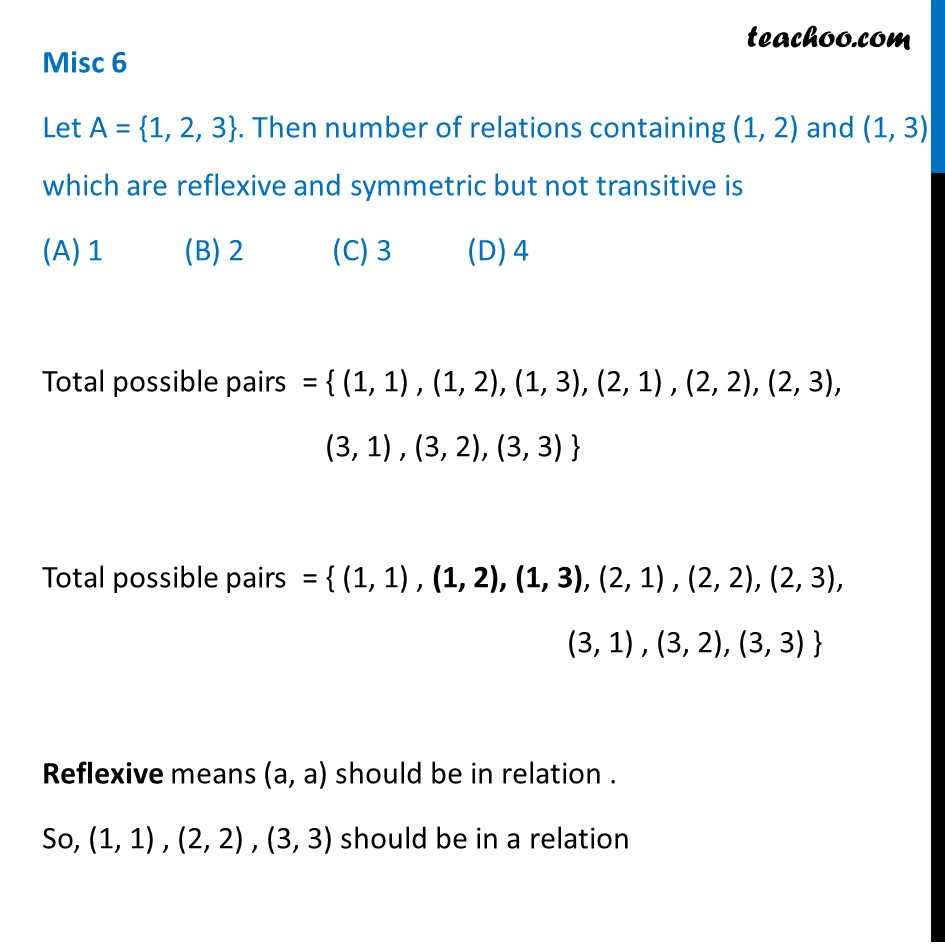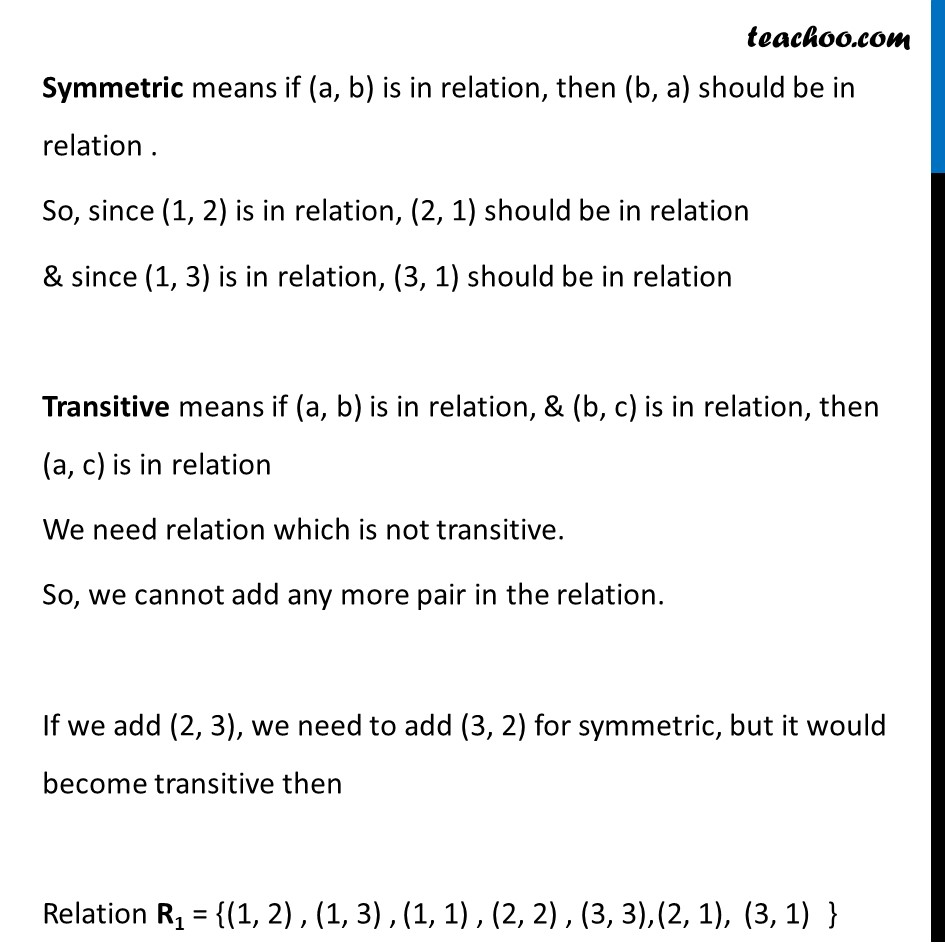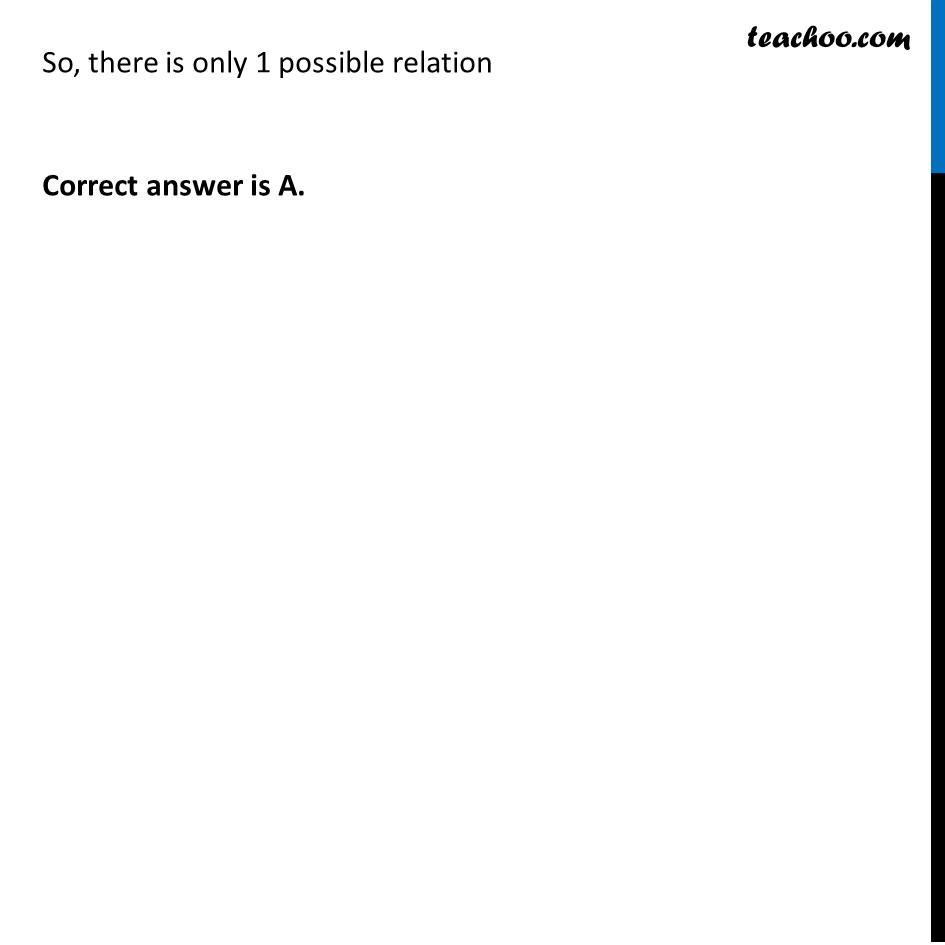Miscellaneous

Chapter 1 Class 12 Relation and Functions
Serial order wiseLearn in your speed, with individual attention - Teachoo Maths 1-on-1 Class

### Transcript

Misc 6 Let A = {1, 2, 3}. Then number of relations containing (1, 2) and (1, 3) which are reflexive and symmetric but not transitive is (A) 1 (B) 2 (C) 3 (D) 4 Total possible pairs = { (1, 1) , (1, 2), (1, 3), (2, 1) , (2, 2), (2, 3), (3, 1) , (3, 2), (3, 3) } Total possible pairs = { (1, 1) , (1, 2), (1, 3), (2, 1) , (2, 2), (2, 3), (3, 1) , (3, 2), (3, 3) } Reflexive means (a, a) should be in relation . So, (1, 1) , (2, 2) , (3, 3) should be in a relation Symmetric means if (a, b) is in relation, then (b, a) should be in relation . So, since (1, 2) is in relation, (2, 1) should be in relation & since (1, 3) is in relation, (3, 1) should be in relation Transitive means if (a, b) is in relation, & (b, c) is in relation, then (a, c) is in relation We need relation which is not transitive. So, we cannot add any more pair in the relation. If we add (2, 3), we need to add (3, 2) for symmetric, but it would become transitive then Relation R1 = { So, there is only 1 possible relation Correct answer is A.